1

Python Pandas 資料切割與複製!!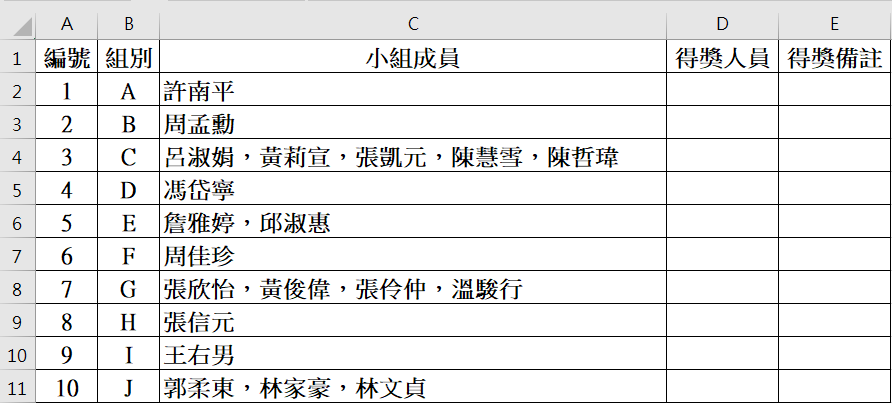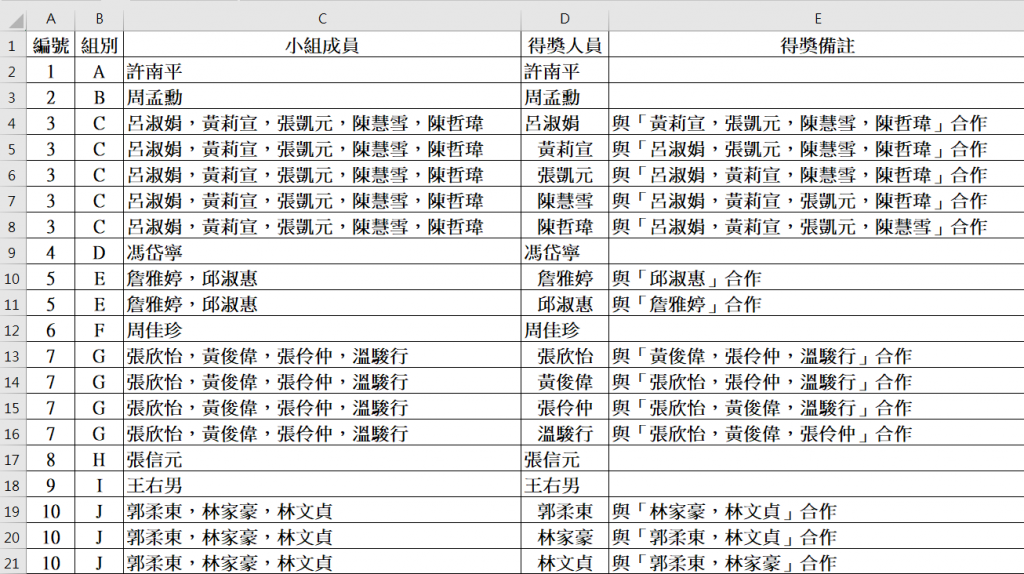import pandas as pd
import numpy as np

Location='sample.xlsx'

df['合作人數'] = df['小組成員'].str.count('，')

df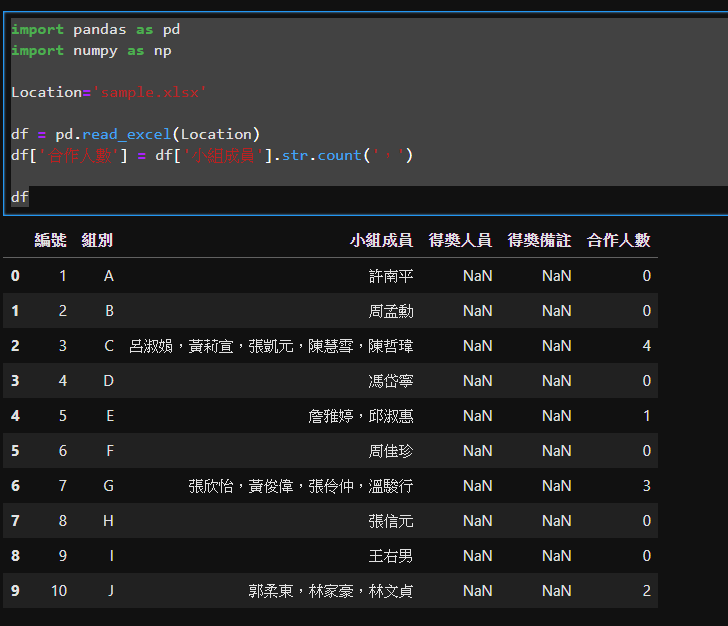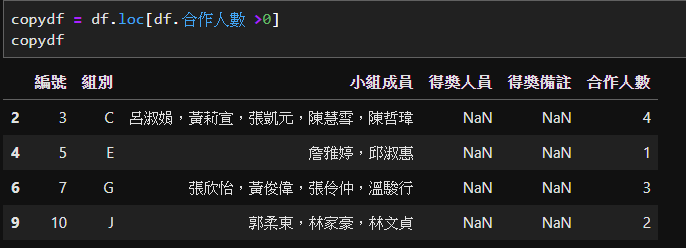lens = df.小組成員.str.split('，').str.len()
df.set_index('組別').reindex(df.組別.repeat(lens)).reset_index()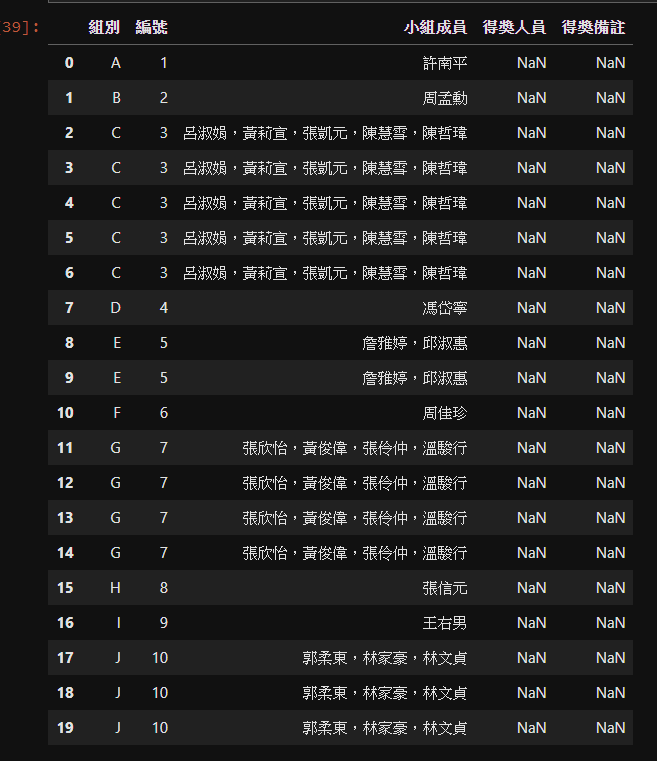ccutmis iT邦高手 4 級 ‧ 2019-10-24 13:03:14 檢舉

http://www.web3d.url.tw/ITHELP/tmp/pandasTest.png

1 個回答

2
import pandas as pd
import numpy as np

def memberWithoutMe(member,me):
tmpStr = '與「'
count = 0
for i in member:
if i != me:
if count>0:
tmpStr = tmpStr + "，"
tmpStr = tmpStr + i
count=count+1
tmpStr = tmpStr + '」合作'
return tmpStr

Location='sample.xlsx'

new_sample0=df[~df['小組成員'].str.contains("，")]
#小組成員只有一人的先撈出來並處理nan及得獎人員欄位
new_sample0=new_sample0.replace(np.nan, '', regex=True)
new_sample0['得獎人員']=new_sample0['小組成員']

df_hasMembers = df[df['小組成員'].str.count('，')>0]
#小組成員不只一人的撈出來跑廻圈處理，以下是廻圈範例:
tmp=[]
for index, row in df_hasMembers.iterrows():
tmpSN=row['編號']
tmpGrp=row['組別']
tmpMember=str(row['小組成員']).split("，")
for i in tmpMember:
tmp.append({
'編號':tmpSN, '組別':tmpGrp,
'小組成員':row['小組成員'],
'得獎人員':i,
'得獎備註':memberWithoutMe(tmpMember,i)
})
result=new_sample0.append(pd.DataFrame(tmp))
print(result.sort_values(by=['組別','編號']))